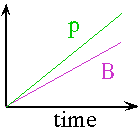Repetitive Acceleration: Cyclic or LinearIn general, static magnetic fields are much stronger than static electric fields. The Lorentz electromagnetic force law

= q (E + v x B)    (SI)

tells us that a 1 Tesla magnetic field exerts the same force on a relativistic particle (v@c) as a 3x108 Volt/metre electric field. This can also be seen by looking that the units, i.e.

1 Volt/metre = 1 (metre/second)Tesla

Using the conversion constant c = 3x108 m/s, we have 1m/s = 1/(3x108), so

3x108 Volt/metre = 1 Tesla

It is easy to generate a 10T (= 3x103 MV/m) static magnetic fields, but static electric fields above about 3 MV/m are almost impossible. This is because our world is made from particles with electric charge but no magnetic charge, so high electric fields are shorted out by sparks consisting of electrically charged particles. There is no known reason why magnetic charges ("magnetic monopoles") should not exist, and they probably do but very rarely and with masses of the order of 1015 GeV/c2.

Sychrotrons

• ring of magnets
• small amount of radiofrequency (r.f.) acceleration
• magnetic field increases synchronously with beam momentum,

B = p/(|q|r)Example: Fermilab Tevatron

• r = 1 km
• B = 0 - 5 Tesla
• DE/orbit ~ 3 MeV    (10m @ 1/3 MV/m)

Þ Efinal = 0.9 TeV   (protons, antiprotons)

 G = final particle energy length of accelerator

for a synchrotron

 G = Emax/2pr @ pmaxc/2pr   (E>>m) \ G (J/m) = |q|rBmaxc/2pr   (SI) = |q|cBmax/2p \ G (eV/m) = |q/e|cBmax/2p   (1.6x10-19 = C/e = J/eV) G = (48 MeV/Tesla)·Bmax

e.g.

Tevatron:  G ~ 150 MeV/m,

LHC:     G ~ 250 MeV/m

Limits to circular accelerators

protons
- size (\$\$)
- B (Hc, stress µ B2)
- S/N (snew ~ 4p/E2, sstotal ~ 102 mb)

electrons

energy loss / orbit
= (4
p/3) e2 b2 g4/r
= 88.5
x10-6 [E(GeV)]4/r(m)

e.g. 88.5 TeV/orbit if E=1TeV and r=1km

Acceleration by Electromagnetic Waves

Power per unit area of an electromagnetic wave (Poynting Vector)

P = ExB/m0

In vacuum: Emax=cBmax, but E & B are 90° out of phase, so

Prms = (Erms)2/cm0

\ Emax = 21/2 Erms = (2Prmsm0c)1/2

So the maximum electric field is

Emax(V/m) = 27.5[Prms(W/m2)]1/2

e.g. 1020 W/m2 Þ Emax= 275 GV/m

But in free space E is transverse to the direction of propagation of the wave, so there can be no continuous acceleration, just oscillation.

Linear Acceleratione.g. SLAC (e± linac)

l = 10.5 cm (3Ghz)
G = 17 MeV/m
L = 3 km
Efinal = 50 GeV

Fermilab proton linac injector

Typical limits on accelerating gradients for copper structures (e.g. SLAC disk-loaded waveguide).c.f. static breakdown voltage of dry air = 3 MV/m